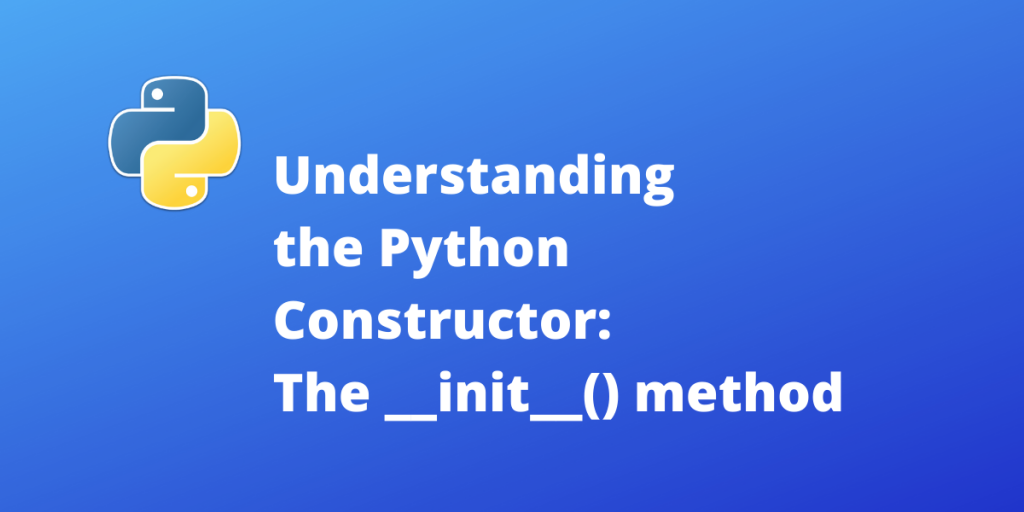# Understanding the __init__() method in PythonIn this article, we discuss a concept of OOPs – The Python Constructor and how explain in detail how we can use __init__() method for initializing an object.

## What is a constructor?

Before we go into the idea of constructor, here is a quick heads up on classes and objects in general:

“In OOP, object refers to an abstract data type created by a developer. An object is defined/characterized by it’s parameters(state-values) and behaviour(methods). Classes are a blueprint or a set of instructions to build a specific type of object.”

Constructors can very simply be understood as a special method that is called during the object initialization/creation. The constructor is usually defined as a function in the class definition that takes in the state parameters and creates an object with those user-defined parameters.

In python the constructor method is __init__(), and is written as:

```def __init__(self, object_parameters...):
# Initialize the object
```

The function takes in self and the object parameters as input. The object parameters as the name suggests are the state variables that define the object.

The ‘self’ is a reserved keyword in python which represents the instance of the class. The ‘self’ keyword allows easy access to the class methods and parameters for use in other methods of the class.

For example, An object state variable ‘var’ can be accessed using self.var.

This idea will become more clear when we see the example of creating a class.

## Creating a simple class: Cartesian Point

In this example we create a class for the class for the 2D Cartesian points. The class has two state variables – x and y which define the point’s location.

```class Point:
def __init__(self, x, y):
self.x = x
self.y = y
def coords(self):
print("The point is at: ({}, {})".format(self.x, self.y))
```

The __init__() method takes self along with the two state variables as input. Then we initialise the object by setting the state variables of the object to the user-defined value.

The state variables of the object( here x and y) can be accessed using self.x and self.y respectively.

The other method here is the coords() that prints the current location of the point. Notice how we access the points using the self.x and self.y.

```# Create object `P` of class Point with value 3 and 4
P = Point1(3, 4)

# Print the coordinates of point `P`
P.coords()
```

## Default Variables in the __init__() method

Like any other function the in python the __init__() method allows you to take default values. This feature can be particularly useful when a class takes in a lot of input parameters. Here we construct another class Point1 which initializes the point to (0, 0) is no data point is specified.

```class Point1:
def __init__(self, x=0, y=0):
self.x = x
self.y = y
def coords(self):
print("The point is at: ({}, {})".format(self.x, self.y))

# Create object of class Point1 with default parameters
Q = Point1()
Q.coords()
```

## Conclusion

We have seen how to define the constructors for a python class. Though is the most viable and easy method, turns out this is not the only method. Python allows more complicated class initialization, which especially becomes essential during inheriting/extending other classes. We will see more of such examples in future articles.